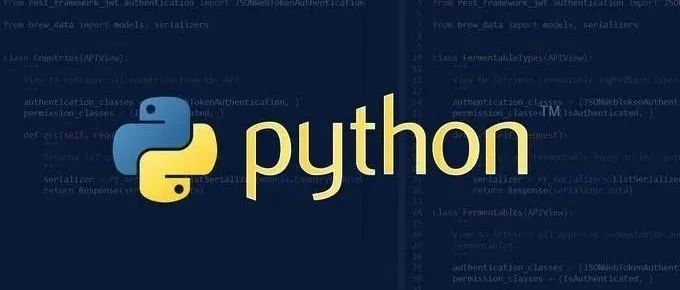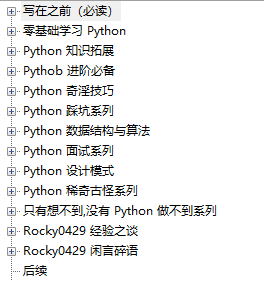# 瞧瞧，这样的代码才叫 Pythonic

10/30 11:30

``加个“星标”，每天一起快乐的学习``Python由于语言的简洁性，让我们以人类思考的方式来写代码，新手更容易上手，老鸟更爱不释手。

## 01. 变量交换

``tmp = aa = bb = tmp``

``a,b = b,a``

## 02. 列表推导

``my_list = []for i in range(10):    my_list.append(i*2)``

``my_list = [i*2 for i in range(10)]``

## 03. 单行表达式

``print('hello'); print('world')if x == 1: print('hello,world')if <complex comparison> and <other complex comparison>:    # do something``

``print（'hello'）print（'world'）if x == 1:    print('hello,world')cond1 = <complex comparison>cond2 = <other complex comparison>if cond1 and cond2:    # do something``

## 04. 带索引遍历

``for i in range(len(my_list)):    print(i, "-->", my_list[i])``

``for i,item in enumerate(my_list):    print(i, "-->",item)``

## 05. 序列解包

``a, *rest = [1, 2, 3]# a = 1, rest = [2, 3]a, *middle, c = [1, 2, 3, 4]# a = 1, middle = [2, 3], c = 4``

## 06. 字符串拼接

``letters = ['s', 'p', 'a', 'm']s=""for let in letters:    s += let``

``letters = ['s', 'p', 'a', 'm']word = ''.join(letters)``

## 07. 真假判断

``if attr == True:    print('True!')if attr == None:    print('attr is None!')``

``if attr:    print('attr is truthy!')if not attr:    print('attr is falsey!')``

## 08. 访问字典元素

``d = {'hello': 'world'}if d.has_key('hello'):    print(d['hello'])    # prints 'world'else:    print('default_value')``

``d = {'hello': 'world'}print(d.get('hello', 'default_value')) # prints 'world'print(d.get('thingy', 'default_value')) # prints 'default_value'``

## 09. 操作列表

``a = [3, 4, 5]b = []for i in a:    if i > 4:        b.append(i)``

``a = [3, 4, 5]b = [i for i in a if i > 4]# Or:b = filter(lambda x: x > 4, a)``

``a = [3, 4, 5]b = map(lambda i: i + 3, a)# b: [6,7,8]``

## 10. 文件读取

``fp = open('file.txt')print(fp.read())fp.close()``

``with open('file.txt') as fp:    for line in fp.readlines():        print(line)``

## 11. 代码续行

``long_string = 'For a long time I used to go to bed early. Sometimes, when I had put out my candle, my eyes would close so quickly that I had not even time to say “I’m going to sleep.”'``

``long_string = 'For a long time I used to go to bed early. ' \              'Sometimes, when I had put out my candle, ' \              'my eyes would close so quickly that I had not even time to say “I’m going to sleep.”'``

``long_string = (    "For a long time I used to go to bed early. Sometimes, "    "when I had put out my candle, my eyes would close so quickly "    "that I had not even time to say “I’m going to sleep.”")``

``from some.deep.module.inside.a.module import (    a_nice_function, another_nice_function, yet_another_nice_function)``

## 12. 显式代码

``def make_complex(*args):    x, y = args    return dict(**locals())``

``def make_complex(x, y):    return {'x': x, 'y': y}``

## 13. 使用占位符

``filename = 'foobar.txt'basename, _, ext = filename.rpartition('.')``

## 14. 链式比较

``score = 85if score > 80 and score < 90:    print("良好")``

``score = 85if 80 < score < 90:    print("良好")``

``>>> False == False == True False``

## 15. 三目运算

``age = 20if age > 18:    type = "adult"else:    type = "teenager"``

``age = 20  b = "adult" if age > 18 else "teenager"````````我将自己的原创文章整理成了一本电子书，取名《Python修炼之道》，一共 400 页，17w+ 字，目录如下：现在免费送给大家，在我的公众号后台回复 修炼之道 即可获取。最后我最近建了一个读者交流群，想加入的可以在公众号后台回复「加群」即可～

推荐阅读：

爆肝整理 400 页 《Python 修炼之道》，高清电子书送给一直支持我的读者！

GitHub 标星 1.6w+，程序员不得不知的“潜规则”又火了！

打脸！这款百度的产品，良心的很不百度

真香！我挖到了一款超级强大的 SQL 工具！

这个插件，牛逼！👆扫描上方二维码即可关注``````

0
0 收藏

### 作者的其它热门文章0 评论
0 收藏
0